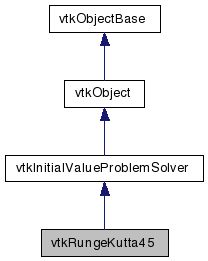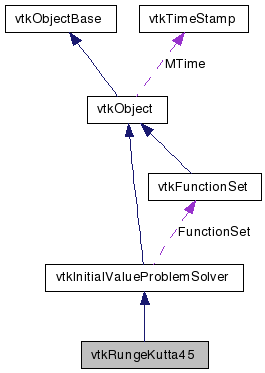# vtkRungeKutta45 Class Reference

`#include <vtkRungeKutta45.h>`

Inheritance diagram for vtkRungeKutta45:[legend]
Collaboration diagram for vtkRungeKutta45:[legend]

## Detailed Description

Integrate an initial value problem using 5th order Runge-Kutta method with adaptive stepsize control.

This is a concrete sub-class of vtkInitialValueProblemSolver. It uses a 5th order Runge-Kutta method with stepsize control to obtain the values of a set of functions at the next time step. The stepsize is adjusted by calculating an estimated error using an embedded 4th order Runge-Kutta formula: Press, W. H. et al., 1992, Numerical Recipes in Fortran, Second Edition, Cambridge University Press Cash, J.R. and Karp, A.H. 1990, ACM Transactions on Mathematical Software, vol 16, pp 201-222

vtkInitialValueProblemSolver vtkRungeKutta4 vtkRungeKutta2 vtkFunctionSet
Tests:
vtkRungeKutta45 (Tests)

Definition at line 42 of file vtkRungeKutta45.h.

## Public Types

typedef
vtkInitialValueProblemSolver
Superclass

## Public Member Functions

virtual const char * GetClassName ()
virtual int IsA (const char *type)
void PrintSelf (ostream &os, vtkIndent indent)
virtual int ComputeNextStep (double *xprev, double *xnext, double t, double &delT, double maxError, double &error)
virtual int ComputeNextStep (double *xprev, double *dxprev, double *xnext, double t, double &delT, double maxError, double &error)
virtual int ComputeNextStep (double *xprev, double *xnext, double t, double &delT, double &delTActual, double minStep, double maxStep, double maxError, double &error)
virtual int ComputeNextStep (double *xprev, double *dxprev, double *xnext, double t, double &delT, double &delTActual, double minStep, double maxStep, double maxError, double &error)

## Static Public Member Functions

static int IsTypeOf (const char *type)
static vtkRungeKutta45SafeDownCast (vtkObject *o)
static vtkRungeKutta45New ()

## Protected Member Functions

vtkRungeKutta45 ()
~vtkRungeKutta45 ()
virtual void Initialize ()
int ComputeAStep (double *xprev, double *dxprev, double *xnext, double t, double &delT, double &error)

## Protected Attributes

double * NextDerivs 

## Static Protected Attributes

static double A 
static double B 
static double C 
static double DC 

## Member Typedef Documentation

Reimplemented from vtkInitialValueProblemSolver.

Definition at line 45 of file vtkRungeKutta45.h.

## Constructor & Destructor Documentation

 vtkRungeKutta45::vtkRungeKutta45 ( ) ` [protected]`

 vtkRungeKutta45::~vtkRungeKutta45 ( ) ` [protected]`

## Member Function Documentation

 virtual const char* vtkRungeKutta45::GetClassName ( ) ` [virtual]`

Reimplemented from vtkInitialValueProblemSolver.

 static int vtkRungeKutta45::IsTypeOf ( const char * name ) ` [static]`

Return 1 if this class type is the same type of (or a subclass of) the named class. Returns 0 otherwise. This method works in combination with vtkTypeRevisionMacro found in vtkSetGet.h.

Reimplemented from vtkInitialValueProblemSolver.

 virtual int vtkRungeKutta45::IsA ( const char * name ) ` [virtual]`

Return 1 if this class is the same type of (or a subclass of) the named class. Returns 0 otherwise. This method works in combination with vtkTypeRevisionMacro found in vtkSetGet.h.

Reimplemented from vtkInitialValueProblemSolver.

 static vtkRungeKutta45* vtkRungeKutta45::SafeDownCast ( vtkObject * o ) ` [static]`

Reimplemented from vtkInitialValueProblemSolver.

 void vtkRungeKutta45::PrintSelf ( ostream & os, vtkIndent indent ) ` [virtual]`

Methods invoked by print to print information about the object including superclasses. Typically not called by the user (use Print() instead) but used in the hierarchical print process to combine the output of several classes.

Reimplemented from vtkInitialValueProblemSolver.

 static vtkRungeKutta45* vtkRungeKutta45::New ( ) ` [static]`

Construct a vtkRungeKutta45 with no initial FunctionSet.

Reimplemented from vtkObject.

 virtual int vtkRungeKutta45::ComputeNextStep ( double * xprev, double * xnext, double t, double & delT, double maxError, double & error ) ` [inline, virtual]`

Given initial values, xprev , initial time, t and a requested time interval, delT calculate values of x at t+delTActual (xnext). Possibly delTActual != delT. This may occur because this solver supports adaptive stepsize control. It tries to change to stepsize such that the (estimated) error of the integration is less than maxError. The solver will not set the stepsize smaller than minStep or larger than maxStep (note that maxStep and minStep should both be positive, whereas delT can be negative). Also note that delT is an in/out argument. vtkRungeKutta45 will modify delT to reflect the best (estimated) size for the next integration step. An estimated value for the error is returned (by reference) in error. This is the norm of the error vector if there are more than one function to be integrated. This method returns an error code representing the nature of the failure: OutOfDomain = 1, NotInitialized = 2, UnexpectedValue = 3

Reimplemented from vtkInitialValueProblemSolver.

Definition at line 67 of file vtkRungeKutta45.h.

 virtual int vtkRungeKutta45::ComputeNextStep ( double * xprev, double * dxprev, double * xnext, double t, double & delT, double maxError, double & error ) ` [inline, virtual]`

Given initial values, xprev , initial time, t and a requested time interval, delT calculate values of x at t+delTActual (xnext). Possibly delTActual != delT. This may occur because this solver supports adaptive stepsize control. It tries to change to stepsize such that the (estimated) error of the integration is less than maxError. The solver will not set the stepsize smaller than minStep or larger than maxStep (note that maxStep and minStep should both be positive, whereas delT can be negative). Also note that delT is an in/out argument. vtkRungeKutta45 will modify delT to reflect the best (estimated) size for the next integration step. An estimated value for the error is returned (by reference) in error. This is the norm of the error vector if there are more than one function to be integrated. This method returns an error code representing the nature of the failure: OutOfDomain = 1, NotInitialized = 2, UnexpectedValue = 3

Reimplemented from vtkInitialValueProblemSolver.

Definition at line 76 of file vtkRungeKutta45.h.

 virtual int vtkRungeKutta45::ComputeNextStep ( double * xprev, double * xnext, double t, double & delT, double & delTActual, double minStep, double maxStep, double maxError, double & error ) ` [inline, virtual]`

Given initial values, xprev , initial time, t and a requested time interval, delT calculate values of x at t+delTActual (xnext). Possibly delTActual != delT. This may occur because this solver supports adaptive stepsize control. It tries to change to stepsize such that the (estimated) error of the integration is less than maxError. The solver will not set the stepsize smaller than minStep or larger than maxStep (note that maxStep and minStep should both be positive, whereas delT can be negative). Also note that delT is an in/out argument. vtkRungeKutta45 will modify delT to reflect the best (estimated) size for the next integration step. An estimated value for the error is returned (by reference) in error. This is the norm of the error vector if there are more than one function to be integrated. This method returns an error code representing the nature of the failure: OutOfDomain = 1, NotInitialized = 2, UnexpectedValue = 3

Reimplemented from vtkInitialValueProblemSolver.

Definition at line 86 of file vtkRungeKutta45.h.

 virtual int vtkRungeKutta45::ComputeNextStep ( double * xprev, double * dxprev, double * xnext, double t, double & delT, double & delTActual, double minStep, double maxStep, double maxError, double & error ) ` [virtual]`

Given initial values, xprev , initial time, t and a requested time interval, delT calculate values of x at t+delTActual (xnext). Possibly delTActual != delT. This may occur because this solver supports adaptive stepsize control. It tries to change to stepsize such that the (estimated) error of the integration is less than maxError. The solver will not set the stepsize smaller than minStep or larger than maxStep (note that maxStep and minStep should both be positive, whereas delT can be negative). Also note that delT is an in/out argument. vtkRungeKutta45 will modify delT to reflect the best (estimated) size for the next integration step. An estimated value for the error is returned (by reference) in error. This is the norm of the error vector if there are more than one function to be integrated. This method returns an error code representing the nature of the failure: OutOfDomain = 1, NotInitialized = 2, UnexpectedValue = 3

Implements vtkInitialValueProblemSolver.

 virtual void vtkRungeKutta45::Initialize ( ) ` [protected, virtual]`

Reimplemented from vtkInitialValueProblemSolver.

 int vtkRungeKutta45::ComputeAStep ( double * xprev, double * dxprev, double * xnext, double t, double & delT, double & error ) ` [protected]`

## Member Data Documentation

 double vtkRungeKutta45::A` [static, protected]`

Definition at line 107 of file vtkRungeKutta45.h.

 double vtkRungeKutta45::B` [static, protected]`

Definition at line 108 of file vtkRungeKutta45.h.

 double vtkRungeKutta45::C` [static, protected]`

Definition at line 109 of file vtkRungeKutta45.h.

 double vtkRungeKutta45::DC` [static, protected]`

Definition at line 110 of file vtkRungeKutta45.h.

 double* vtkRungeKutta45::NextDerivs` [protected]`

Definition at line 112 of file vtkRungeKutta45.h.

The documentation for this class was generated from the following file:

Generated on Wed Jun 3 19:32:27 2009 for VTK by1.5.6